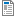# Ordinary Differential Equations

Ordinary Differential Equations

National Taiwan University of Science and Technology

Department of Electrical Engineering

Summer, 2019

# Instructor : Shun-Feng Su,

Office : T2 502-3

Phone: ext 6704

E-mail : sfsu@mail.ntust.edu.tw

Classroom :  TBD

Time : As specifed in the file feasible-schedule-ODE

Textbook :     P.V. O’Neil, Advanced Engineering Mathematics, 7th Edition, Cengage Learning, 2012. (華泰)

Homework: Homework will be given for each section. But it is not necessary to turn in

Tests :          Three Midterms and one final. (25% each)

Ordinary Differential Equations

This a basic course in Engineering. In this course, we shall discuss the fundamental ideas for ordinary differential equations and the ways of solving them. The contents are all basics. In addition to those ideas and methods, I shall also emphasize some theoretical proofs. I hope that students can learn how to prove theorems. Several different ways of theorem proving will be introduced in the class.

Tentative Outline

• Introduction to Differential Equations
• First-Order Differential Equations
• Midterm I
• Linear Equations of Higher Order
• Midterm II
• Laplace Transform
• Midterm III
• Power Series Methods
• Special Function (If time allowed)
• Final

Note:

Attachment:DFi-2019.docDFii-2019.docDFiii-2019.DOCDFfin-2019.DOC